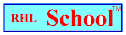Name_______________________________________________Date_________________________
Mathematics Problem Solving
Volume 5, Number 10, November 8, 1999
www.rhlschool.com

### Squared Riddles

When you see a number like this: 52, you read it as five squared (or five to the second power). When a number is squared, it is multiplied times itself. (It does not mean that it’s multiplied by two.)

52 = 25102 = 10092 = 81

The square root of a number is the number that was multiplied times itself to become that number. The square root of 25 is 5. The square root of 100 is 10. The square root of 81 is 9.

The following riddles will give you practice in thinking about and remembering what it means to square a number.

1. I am a number. I am not zero. If I am squared, I’m still the same. What number am I?

2. I am a number. I am the sum of 42 and 62. What number am I?

3. I am a number. I am the square root of the number of squares on a chess or checker board. What number am I?

4. I am a state in New England. If you find the square root of half of 98, you’ll know the number of letters in my name. What state am I?

rhlschool.com

RHL School - Free Worksheets and MoreCopyright 1999 RHL

RHL School Home